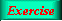Lecture 4 - Page 22 : 34
 Functional Programming in SchemeHigher-order Functions * Introduction to higher-order functions Higher-order functions Some simple and general higher-order functions Linear search in lists Generation of list selectors * Mapping and filtering Classical higher-order functions: Overview Mapping The mapping function Examples of mapping Filtering The filtering function Examples of filtering * Reduction and zipping Reduction The reduction functions Accumulation Zipping The zipping function * Currying The idea of currying Currying in Scheme Examples of currying Ad hoc currying in Scheme (1) Ad hoc currying in Scheme (2) * Web related higher-order functions HTML mirror generation HTML mirror usage examples Making tables with the real mirror Tables with higher-order functions HTML element modifications The function simple-html-table The XHTML mirror in LAML Generation of a leq predicate from enumeration
 Currying in Scheme
 It is possible to generate curried functions in Scheme.But the parenthesis notation of Lisp does not fit very well with the idea of currying
 ```(define (curry2 f) (lambda(x) (lambda(y) (f x y)))) (define (curry3 f) (lambda(x) (lambda(y) (lambda(z) (f x y z))))) (define (uncurry2 f) (lambda (x y) ((f x) y))) (define (uncurry3 f) (lambda (x y z) (((f x) y) z)))```

Generation of curried and uncurried functions in Scheme.Playing with curried functions in Scheme